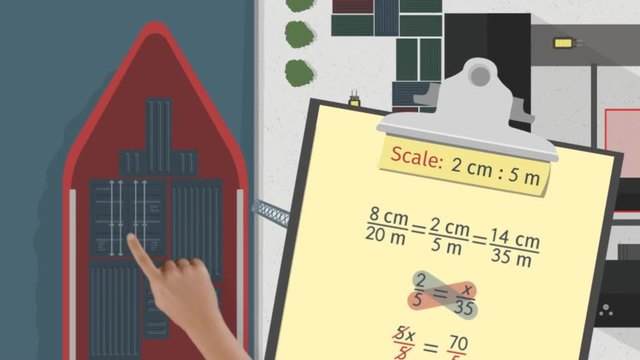# How to Create a Scale DrawingRating

Ø 5.0 / 1 ratings
The authorsTeam Digital

## Basics on the topicHow to Create a Scale Drawing

After this lesson, you will be able to create your own scale drawings of the top-view of a complicated space (e.g. furnished room or building, park, etc.).

The lesson begins by teaching you how to chose a scale factor. It leads you to learn how to set up and solve equivalent ratios. It concludes with converting actual measurements using a scale factor.

Learn about scale drawings by helping Alexey figure out if he can fit all of the cargo into the shipyard.

This video includes key concepts, notation, and vocabulary such as the term scale factor (the ratio of any two corresponding side lengths of two similar geometric objects), scale ratio (a fraction with the scale drawing as the numerator and actual object as the denominator), and equivalent ratios (two ratios that express the same relationship).

Before watching this video, you should already be familiar with rotations, reflections, and packing problems that can be visualized as fitting puzzle or Tetris pieces in a small space.

After watching this video, you will be prepared to learn about 3D scale drawings.

Common Core Standard(s) in focus: 7.G.A.1 A video intended for math students in the 7th grade Recommended for students who are 12 - 13 years old

### TranscriptHow to Create a Scale Drawing

Alexey works in the busiest shipyard in all of Russia. He's an expert at organizing cargo of all shapes and sizes. A ship has just arrived at the docks. It has so much cargo that it is hard to tell if it can all fit in the yard! Alexey decides to create a scale drawing to help him figure out if he can fit all the cargo into the shipyard. The shipyard is 20 meters by 35 meters. To make a scale drawing, we first need to choose a scale factor. Remember, a scale factor is the ratio of any two corresponding side lengths of two similar geometric objects, like the scale drawing of the shipyard and the actual shipyard. Let's choose a scale factor of 2 centimeters in the drawing for every 5 meters in the actual shipyard. To rewrite our scale factor as a fraction, we always take the scale drawing as the numerator and the actual object as the denominator. We would like to use it to figure out how many centimeters in the drawing represents 20 meters in the actual shipyard and how many centimeters in the drawing represents 35 meters. Since we want the scale drawing and the actual shipyard to be proportional to each other, we would like these ratios to be equivalent ratios, meaning that they are all equal to each other. To find out how many centimeters represents 20 meters, we take the first two ratios and let our unknown be 'x', giving us the following equation. Cross-multiplying and solving for 'x', we find that 8 centimeters in the scale drawing represents 20 meters in the actual shipyard. Similarly, we take the last two ratios to figure out how many centimeters represents 35 meters. This gives us an equation. Cross-multiplying and solving for 'x', we get that 14 centimenters represents 35 meters in Alexey's scale drawing. So the dimensions of the shipyard in the drawing are 8 centimeters by 14 centimeters. Now Alexey is ready to pack in some cargo! Looks like the cargo consists of box crates, rectangular containers, and T-shaped cargo? First, the box crates. The crates are 7.5 meters wide and 7.5 meters long. As before, rewriting the scale factor as a fraction, we want to figure out how many centimeters in the scale drawing represents 7.5 meters. As we want the scale drawing of the box crates to be proportional to the actual box crates, we set these ratios equal to each other to indicate that they are equivalent ratios. Just as before, we have an equation. Cross-multiply and solve for our unknown to get that the square containers are 3 centimeters wide and 3 centimeters long in Alexey's scale drawing. It's time to place the box crates! Nice work, Alexey! Now Alexey needs to pack the rectangular containers into the shipyard. The containers are 10 meters long and 5 meters wide. How can we draw these containers? One length is covered, as we know from our scale factor that 2 centimeters represents 5 meters. We need to figure out how many centimeters represents 10 meters. We can set them equal to each other to get an equation. Cross-multiplying and solving for 'x', gives us 4 centimeters for every 10 meters. So each container will be 2 centimeters wide and 4 centimeters long. Whoa, that's a tight fit! Now for the T-shaped cargo.What is in these anyway? In real life, the actual sides of this cargo measure 2.5 meters, 5 meters, and 7.5 meters. How much space will these take up in Alexey's drawing? One length is covered, as we know from our scale factor that 5 meters is represented by 2 centimeters. We also know from our calculations with the box crates that 7.5 meters is represented by 3 centimeters. So all that is left is to find out how many centimeters represents 2.5 meters. Setting up an equation. Cross-multiplying and solving for 'x', gives us 1 centimeter for every 2.5 meters. As for arranging the cargo, they all just barely fit! Let's review our methods. First, choose the scale factor of our scale drawing. Then we note the actual measurements of the object we want to draw. Then we convert the actual measurements using our scale factor. We do this by setting up an equation of equivalent ratios, cross-multiplying, and solving for the unknown. Finally, we have what we need to create a scale drawing! After a hard day's work, Alexey can kick back and relax with his favorite video game. Hmm, looks like Alexey has a one-track mind!